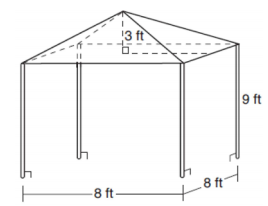mycollegehive
A vendor is using an 8-ft by 8-ft tent for a craft fair. The legs of the tent are 9 ft tall and the top forms a square pyramid with a height of 3 ft. What is the volume, in cubic feet, of space the tent occupies?
JMAP geometry math pyramis prism0A vendor is using an 8-ft by 8-ft tent for a craft fair. The legs of the tent are 9 ft tall and the top forms a square pyramid with a height of 3 ft.What is the volume, in cubic feet, of space the tent occupies?

1) 256

2) 640

3) 672

4) 768

54 viewsShareFollowUniversity of Lagos Nigeria
23 June 2020University of Benin Nigeria
23 June 20200The diagram above comprises of a square base pyramid (at the top) and a square base prims (below).

Thus, the total volume = Volume of pyramid + Volume of prism

Volume of pyramid = 1/3 x Ah

Where A and h are the base area and height of the pyramid respectively.

Since the base is a square, A = Length x Width

A = 8 x 8 = 64

Therefore, Volume of pyramid = 1/3 x 64 x 3

= 64

Volume of prims = L x W x H

Where L, W and H are the Length, Width and Hight of the square base prism respectively

Volume of prism = 8 x 8 x 9

= 576.

Thus, total volume = 64 + 576

= 640

Ans 2Share### Related Tags

JMAP

2 followers

141 questionsgeometry

2 followers

99 questionsmath

2 followers

990 questions